# Electronics and Communication Engineering - Exam Questions Papers

21.

A uniform plane magnetic wave incident normally on a plane surface of a dielectric material is reflected and the percentage of reflected power is 75% What is VSWR?

 A. 14 B. 1.4 C. 15 D. 13

Explanation: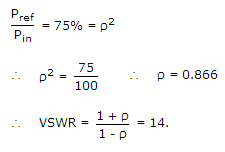22.

Silicon is doped with boron to a concentration of 4 x 1017 atoms/cm3. Assuming the intrinsic carrier concentration of silicon to be 1.5 x 1010/cm3 and the value of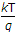to be 25mV at 300K. Compared to undoped silicon, the Fermi level of doped silicon

 A. goes down by 0.13 eV B. goes up by 0.13 eV C. goes down by 0.427 eV D. goes up by 0.427 eV

Explanation:

Use EF - Ev =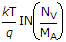Since it is doped with acceptor impurity, Fermi level will shift down.

23.

The Fourier series of a real periodic function has only

1. Cosine terms if it is even
2. Sine terms if it is even
3. Cosine terms if it is odd
4. Sine terms if it is odd
Which of the above statements are correct?

 A. (1) and (4) B. (1) and (2) C. (2) and (4) D. (2) and (3)

Explanation: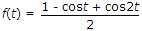, then it has 0 and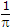HZ frequency component.

24.

A communication channel with AWGN operating at a signal to noise ratio SNR >> 1 and bandwidth B has capacity C1. If the SNR is doubled keeping B constant, the resulting capacity C2 is given by

 A. C2 ≈ 2C1 B. C2 ≈ C1 + B C. C2 ≈ C1 + 2B D. C2 ≈ C1 + 0.3B

Explanation:

C = B log2[1 + SNR]

C = B log2[SNR]

C' = B log2[2SNR] = B log2 SNR + B log22

C' = C1 + B.

25.

Power gain of antenna equals directive gain in VHF and UHF range if and only if efficiency of the antenna is __________ .

 A. 0.25 B. 0.5 C. 0.75 D. 1

Explanation:

Directive gain and power gain are identical except that power gain takes into account the antenna losses. It may be written as

Gp = ηGd η => efficiency

If η = 1 , GP = Gd .

#### Current Affairs 2022

Interview Questions and Answers